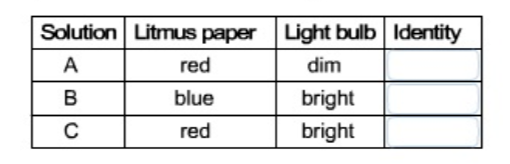# Problem: You have three solutions: HI(ag), HCN(ag), and KOH(ag), but you don't know which is which, so you test each with litmus paper. In addition, you use each solution to complete the electrical circuit for a light bulb. The results are are shown in the table below.Complete this table with the identity of each solution.

###### FREE Expert Solution###### Problem Details

You have three solutions: HI(ag), HCN(ag), and KOH(ag), but you don't know which is which, so you test each with litmus paper. In addition, you use each solution to complete the electrical circuit for a light bulb. The results are are shown in the table below.

Complete this table with the identity of each solution.What scientific concept do you need to know in order to solve this problem?

Our tutors have indicated that to solve this problem you will need to apply the Acid Base Indicators concept. If you need more Acid Base Indicators practice, you can also practice Acid Base Indicators practice problems.

What is the difficulty of this problem?

Our tutors rated the difficulty ofYou have three solutions: HI(ag), HCN(ag), and KOH(ag), but ...as low difficulty.

How long does this problem take to solve?

Our expert Chemistry tutor, Dasha took 3 minutes and 22 seconds to solve this problem. You can follow their steps in the video explanation above.

What professor is this problem relevant for?

Based on our data, we think this problem is relevant for Professor McVey's class at TXSTATE.